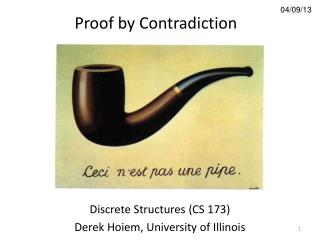DownloadDownload Presentation04/09/13

# 04/09/13

Télécharger la présentation## 04/09/13

- - - - - - - - - - - - - - - - - - - - - - - - - - - E N D - - - - - - - - - - - - - - - - - - - - - - - - - - -
##### Presentation Transcript

1. Proof by Contradiction 04/09/13 Discrete Structures (CS 173) Derek Hoiem, University of Illinois

2. Administrative • Homeworks due Wed • Tests released during discussion sections • Grades released shortly before (Wed at earliest) • Honors HW 2 posted (due Apr 29)

3. About conflict exams for final • Look for posted conflict exams that might help out. These are largely from courses with combined exams. CS 173, like most non-combined exams, doesn't have one. • Two exams at the same time? The course with the combined exam handles it. (Or, if there's more than one, the largest such course.) • Three exams in a row? The largest course handles it. • Really large combined exams often have an unposted "conflict conflict" time already set up, that you'll only find out about after you report a conflict to their course staff. • Conflicts must be reported to instructors by the last day of classes. Earlier is better. If you aren't 100% sure which course is handling it, notify all the instructors involved, so we can help make sure you end up with a solution. • More details are on the student code exams page

4. Last class • Analysis of running time of code • P vs. NP: Problems in NP can be verified in polynomial time. Problems in P can also be solved in polynomial time. • This class • Proof by contradiction • When to use different types of proofs

5. Proof by Contradiction • Sometimes you want to show that something is impossible • cannot be written as a ratio of integers • There is no compression algorithm that reduces the size of all files • A cycle with an odd number of nodes can’t be colored with two colors • Difficult to prove non-existence directly, and can’t prove by example • Solution: show that the negation of the claim leads to a contradiction

6. Contradiction A set of propositions is a contradiction if their conjunction is always false Contradiction? and is odd is negative number and is real

7. Proof by contradiction Claim: There are infinitely many prime numbers Equivalent claim: There is not a finite set of primes.

8. Basic form of proof by contradiction • I need to show proposition • Suppose, instead, that is false. • Then, we can see that both and, which is a contradiction. • Therefore, must be true.

9. Why proof by contradiction works We need to prove proposition Instead, we show , i.e., that we can conclude a contradiction from not By contrapositive,

10. Another explanation We need to prove proposition Instead, we show , i.e., that we can conclude a contradiction from But means that , so

12. Proof by contradiction Claim: is irrational Equivalent claim: There does not exist a pair of integers without common factors such that

13. Proof by contradiction Claim: No lossless compression algorithm can reduce the size of every file.

14. Why image compression works: images are mostly smooth

15. Lossless compression (PNG) • Predict that a pixel’s value based on its upper-left neighborhood • Store difference of predicted and actual value • Pkzip it (DEFLATE algorithm)

16. Proof by contradiction Claim: A cycle graph with an odd number of nodes is not 2-colorable.

17. Proof by contradiction or contrapositive Claim: For all integers , if is odd, then is odd.

18. When to use each type of proof Match the situation to the proof type Situation • Can see how conclusion directly follows from hypothesis • Need to demonstrate claim for an unbounded set of integers • Easier to show that negation of hypothesis follows from negation of conclusion • Need to show that something doesn’t exist • Need to show that something exists Proof type • Direct proof • Proof by example or counter-example • Proof by contrapositive (or logical equivalence) • Induction • Proof by contradiction

19. When to use each type of proof Match the situation to the proof type Situation • Can see how conclusion directly follows from claim (a) • Need to demonstrate claim for an unbounded set of integers (d) • Easier to show that negation of hypothesis follows from negation of conclusion (c) • Need to show that something doesn’t exist (e) • Need to show that something exists (b) Proof type • Direct proof • Proof by example or counter-example • Proof by contrapositive (or logical equivalence) • Induction • Proof by contradiction

20. When to use each type of proof Match the claim to the suitable proof type Claim • There is no real , such that • If is odd, then is odd. • There is an integer , such that . • If is odd, then is odd. • All trees have more nodes than edges. • A wheel graph can’t be colored with two colors. • Not every natural number is a square. • The sum of two rational numbers is rational. • An even number can’t be created from the product of odd numbers. Proof type • Direct proof • Proof by example or counter-example • Proof by contrapositive (or logical equivalence) • Induction • Proof by contradiction

21. Next class • Collections of sets • Sets of sets • Powersets • Partitions